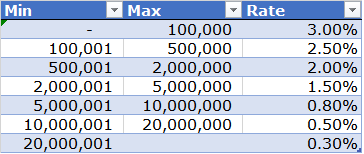Consider a simple dataset as shown below:For each tier, the tier rate is incrementally applied to the volume within the tier volume range.  Given the following transaction volumes, one may want to compute the transaction feeThe expected result is shown belowAs one can observe, for a transaction value of 400,000, the fee has been computed as 3% on the first 100,000 and 2.5% on the next 300,000. You may download my solution workbook from here. In the file, I have shared 2 solutions – a conventional formula based one and a PowerPivot solution.

I have also solved a similar question here.